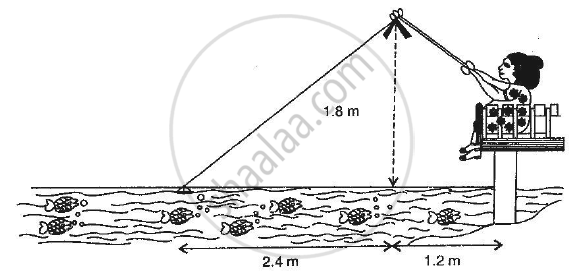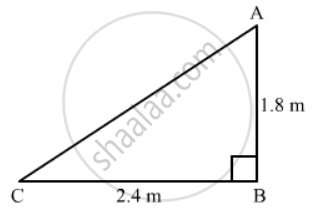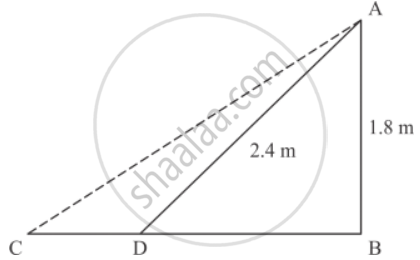Advertisement Remove all ads

# Nazima is Fly Fishing in a Stream. the Tip of Her Fishing Rod is 1.8 M Above the Surface of the Water and the Fly at the End of the String Rests on the Water 3.6 M Away and 2.4 M from a Point Directly - Mathematics

Sum

Nazima is fly fishing in a stream. The tip of her fishing rod is 1.8 m above the surface of the water and the fly at the end of the string rests on the water 3.6 m away and 2.4 m from a point directly under the tip of the road. Assuming that her string (from the tip of her road to the fly) is taut, how much string does she have out (in the given figure)? If she pulls the string at the rate of 5 cm per second, what will the horizontal distance of the fly from her after 12 seconds.Advertisement Remove all ads

#### Solution

Let us take

AB = Tip of fishing rod above the surface of the water

BC = The string rest from directly under the tip of the rod.

AC = The length of stringIn ABC right triangle right angled at B

AC^2=AB^2+BC^2

AC^2=1.8^2+2.4^2

AC^2=3.24+5.76

AC^2=9

AC^2=sqrt9

AC^2sqrt(3xx3)

Hence 3 m string is out

The string pulled in 12 seconds is equal to 12xx5=60cm=0.6m to point

In this case the diagram will look like the followingNow The length of the new string AD = AC − CD = 3.00 − 0.6 = 2.4 m

Now in triangle ADB we have

1.8^2+BD^2=2.4^2

3.24+BD^2=5.76

BD^2=5.76-3.24

BD^2=2.52

BD=1.587

∴ Required distance = 1.587+1.2=2.79 m

Hence, the horizontal distance is 2.97m.

Concept: Triangles Examples and Solutions
Is there an error in this question or solution?
Advertisement Remove all ads

#### APPEARS IN

RD Sharma Class 10 Maths
Chapter 7 Triangles
Q 38 | Page 128
Advertisement Remove all ads

#### Video TutorialsVIEW ALL 

Advertisement Remove all ads
Share
Notifications

View all notifications

Forgot password?
Course